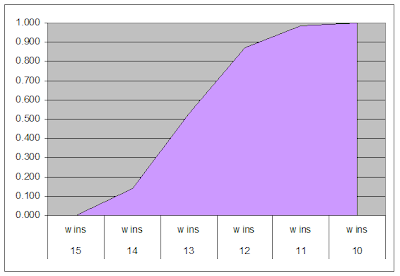## Total Probabilities

In the final stretch of the 2006 regular season I wanted to know which teams would make the playoffs. I thought that as long as my model was somewhat valid, it would give insight on how various teams would finish the season.

As opposed to my original linear model, which predicted the number of total season wins for each team based on to-date efficiency, my new game-by-game model takes matchups and homefield advantage into account. As the number of remaining games dwindle, these factors become more important.

Baltimore is my favorite team, so it was the first one I analyzed. With 5 games remaining in the season, the probability models (both the original (model 1) and alternate (model 2)) showed the following win probabilities for the Ravens.The probabilities for each possible season outcome, i.e. total number of wins can be computed. For example, the probability that the Ravens would end the season by winning all 5 remaining games would be the product of all the individual win probabilities. At this point in the season it was:

Prob(5 wins) = .50 * .48 * .95 * .70 * .91 = .14

Every possible combination of game outcomes was calculated. There were 2^5 possible combinations of outcomes with 5 games remaining. Each combination that leads to 4 wins is summed to give the total probability of winning 4 of the 5 remaining games. The same is done for 3, 2, 1, and 0 wins. With a record of 9-2, the Ravens' resulting probabilities were:The cumulative probabilities of winning "at least" X number of games is easily calculated by adding the probabilities of all the possible outcomes of X and greater than X. Baltimore's probabilities of winning at least X number of games was:As luck would have it, Baltimore indeed finished the season with 13 wins, the number predicted most likely by the model.

These calculations were performed for all 32 teams. Once computed, various teams of interest can be compared. For example, who would win the AFC North? Simply compare BAL's and CIN's total win probabilities. Who would likely win homefield advantage in the playoffs? Compare BAL's and IND's total win predictions.

### Leave a Reply

Note: Only a member of this blog may post a comment.